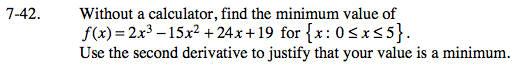### Home > CALC > Chapter Ch7 > Lesson 7.1.4 > Problem7-42

7-42.Find f '(x) and f ''(x).Identify candidates for minimum values by finding where f '(x) = 0 and where f '(x) = DNE. Note: this includes the endpoints.

Evaluate all candidates (except for the endpoints) in the 2nd derivative.
Recall that a LOCAL minimum will exist where f '(candidate) = 0 or DNE and f ''(candidate) > 0.
Note: endpoints can be global (not local) minima.

To find the GLOBAL minimum, evaluate and compare f (local minimum) with f (endpoint) and f (endpoint b).
The lowest value is the global minimum.